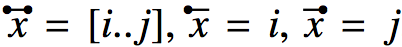Instructors are free to include the exercises from the book in their lecture notes and course assignments. We provide here the LaTeX source code of all exercises in the book, as well as of some additional exercises we devised after publishing.

Chapter 1. Molecular biology and high-throughput sequencing [PDF] [LATEX]
Chapter 2. Algorithm design [PDF] [LATEX]
Chapter 3. Data structures [PDF] [LATEX]
Chapter 4. Graphs [PDF] [LATEX]
Chapter 5. Network flows [PDF] [LATEX]
Chapter 6. Alignments [PDF] [LATEX]
Chapter 7. Hidden Markov models (HMMs) [PDF] [LATEX]
Chapter 8. Classical indexes [PDF] [LATEX]
Chapter 9. Burrows-Wheeler indexes [PDF] [LATEX]
Chapter 10. Read alignment [PDF] [LATEX]
Chapter 11. Genome analysis and comparison [PDF] [LATEX]
Chapter 12. Genome compression [PDF] [LATEX]
Chapter 13. Fragment assembly [PDF] [LATEX]
Chapter 14. Genomics [PDF] [LATEX]
Chapter 15. Transcriptomics [PDF] [LATEX]
Chapter 16. Metagenomics [PDF] [LATEX]

To reproduce the interval notation used in the book, i.e.you can use the commands:
$\INTERVAL{x} = [i..j]$, $\SP{x}=i$, $\EP{x}=j$
which are defined with the following LaTeX macros (see also the LaTeX code of the exercises for Chapter 9):
\makeatletter
\newcommand{\fullbullet}{\begin{picture}(-1,.5)(1,-.2)\circle*{2.1}\end{picture}}

\newcommand\SP{\vbox{\m@th\ialign{##\crcr
\kern.1em\fullbullet\crcr\noalign{\kern-\p@\nointerlineskip}%
$\hfil\displaystyle\overline{#1}\hfil$\crcr}}}

\newcommand\EP{\vbox{\m@th\ialign{##\crcr
\hfill\fullbullet\crcr\noalign{\kern-\p@\nointerlineskip}%
$\hfil\displaystyle\overline{#1}\hfil$\crcr}}}

\newcommand\INTERVAL{\vbox{\m@th\ialign{##\crcr
\kern.1em\fullbullet\hfill\fullbullet\crcr\noalign{\kern-\p@\nointerlineskip}%
$\hfil\displaystyle\overline{#1}\hfil$\crcr}}}
\makeatother